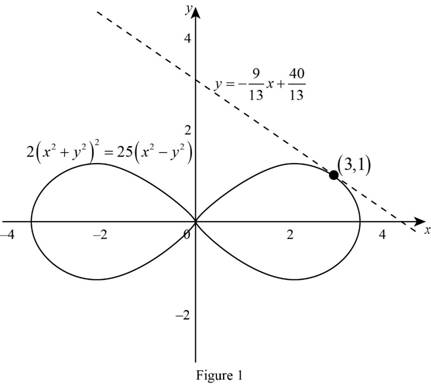# The equation of the tangent line to the given equation at the point.### Single Variable Calculus: Concepts...

4th Edition
James Stewart
Publisher: Cengage Learning
ISBN: 9781337687805### Single Variable Calculus: Concepts...

4th Edition
James Stewart
Publisher: Cengage Learning
ISBN: 9781337687805

#### Solutions

Chapter 3.5, Problem 27E
To determine

## To find: The equation of the tangent line to the given equation at the point.

Expert Solution

The equation of the tangent line to the equation 2(x2+y2)2=25(x2y2) at the point (3,1) is y=913x+4013.

### Explanation of Solution

Given:

The curve is 2(x2+y2)2=25(x2y2).

The point is (3,1).

Derivative rule: Chain rule

If y=f(u) and u=g(x) are both differentiable function, then dydx=dydududx.

Formula used:

The equation of the tangent line at (x1,y1) is, yy1=m(xx1) (1)

Where, m is the slope of the tangent line at (x1,y1) and m=dydx|(x,y)=(x1,y1).

Calculation:

Consider the given equation 2(x2+y2)2=25(x2y2).

Differentiate the given equation implicitly with respect to x.

ddx(2(x2+y2)2)=ddx(25(x2y2))2ddx(x2+y2)2=25ddx(x2y2)

Let u=x2+y2 and  k=x2y2.

Then, 2ddx(u2)=25ddx(v).

Apply the chain rule and simplify the terms.

2[ddu(u2)dudx]=25[ddv(v)dvdx]2[2ududx]=25[1dvdx]4ududx=25dvdx

Substitute u=x2+y2 and  k=x2y2.

4(x2+y2)ddx(x2+y2)=25ddx(x2y2)4(x2+y2)[ddx(x2)+ddx(y2)]=25[ddx(x2)ddx(y2)]4(x2+y2)[2x+ddx(y2)]=25[2xddx(y2)]

Apply the chain rule and simplify the terms.

4(x2+y2)[2x+2ydydx]=25[2x2ydydx]4(x2+y2)[x+ydydx]=25[xydydx]4x(x2+y2)+4y(x2+y2)dydx=25x25ydydx

Combine the terms dydx to one side of the equation.

4y(x2+y2)dydx+25ydydx=25x4x(x2+y2)(4y(x2+y2)+25y)dydx=25x4x(x2+y2)dydx=25x4x(x2+y2)4y(x2+y2)+25y

Therefore, the derivative of the equation is dydx=25x4x(x2+y2)4y(x2+y2)+25y.

The slope of the tangent line at (3,1) is computed as follows,

dydx|(x,y)=(3,1)=25(3)4(3)(32+12)4(1)(32+12)+25(1)=7512(10)4(10)+25=4565=913

Thus, the slope of the tangent line at the point (3,1) is m=913.

Substitute (3,1) for (x1,y1) and m=913 in equation (1).

y1=913(x3)y1=913x+2713y=913x+2713+1y=913x+4013

Therefore, the equation of the tangent line to the equation 2(x2+y2)2=25(x2y2) at the point (3,1) is y=913x+4013.

Graph:

The graph of the given curve and tangent line is shown below Figure 1.From Figure 1, it is observed that the line y=913x+4013 is tangent to the equation 2(x2+y2)2=25(x2y2) at the point at (3,1).

### Have a homework question?

Subscribe to bartleby learn! Ask subject matter experts 30 homework questions each month. Plus, you’ll have access to millions of step-by-step textbook answers!